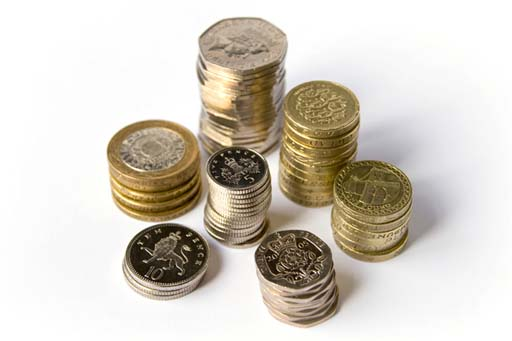### Become an OU studentManaging my money

Start this free course now. Just create an account and sign in. Enrol and complete the course for a free statement of participation or digital badge if available.

# 6.3.2 Targeting the current asset ratio

The household balance sheet can also be used to calculate the current asset ratio. This compares total liquid assets with total short-term liabilities and is useful in assessing how finances can be managed in the short term. It is calculated in the following way:

Current asset ratio = Total liquid assets / Total short-term liabilities

Personal finance experts say that the current assets ratio should always exceed 1. If it is below 1, then that tells us that short-term liabilities (such as bills and credit card debts) could not be met if circumstances made it necessary to do so. This can indicate that potentially serious financial difficulties could lie ahead. The risks are insolvency and potentially even bankruptcy.

Personal finance experts often argue that a good target to aim for – when well into adult life – would be a current asset ratio of 4, though a less conservative target might be only 3.

Now use the figures you’ve produced on your household balance sheet to calculate your current asset ratio by dividing total liquid assets (C) by total short-term liabilities (D). How might this be used in financial planning?

One possible use of your current asset ratio would be to assess the progress of particular financial plans. Someone with the goal of getting out of short-term debt, for example, could calculate their current asset ratio every three months and track it as it (hopefully) moves to a figure above 1.Figure 10